# Trapezium

A trapezium is a convex quadrilateral with exactly one pair of opposite sides parallel to each other. The trapezium is a two-dimensional shape that appears as a table when drawn on a sheet of paper. In Euclidean Geometry, a quadrilateral is defined as a polygon with four sides and four vertices. Hence, a trapezium also has four sides, four angles and four vertices.

There are many examples of trapezium that can be seen in real life. A major application of trapezium is the trapezium rule, where the area under the curve is divided into a number of trapeziums and then the area of each trapezium is evaluated.

Let us learn all the properties of trapezium along with area and perimeter formula, its types and examples.

## What is a Trapezium?

The trapezium is a quadrilateral with one pair of parallel opposite sides. The parallel sides of a trapezium are called bases and the non-parallel sides of a trapezium are called legs. It is also called a trapezoid. Sometimes the parallelogram is also called a trapezoid with two parallel sides.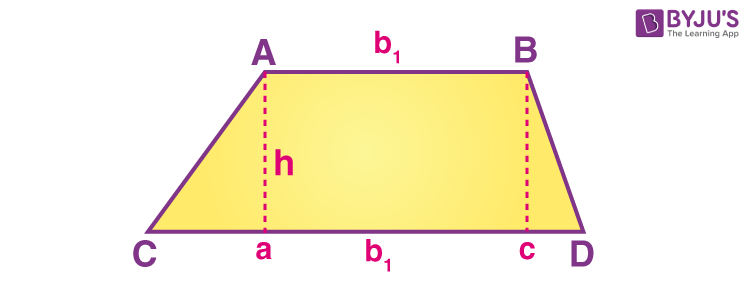From the above figure, we can see, sides AB and CD are parallel to each other whereas AC and BD are non-parallel sides. Also, ‘h’ is the distance between the two parallel sides which demonstrates the height of the trapezium.

### Shape of Trapezium

A trapezium is a closed shape or a polygon, that has four sides, four corners/vertices and four angles. Anyone pair of opposite sides of a trapezium are parallel to each other.

There are many real-life examples of trapezium shape that we can see around us. For example, a table whose surface is shaped like a trapezium.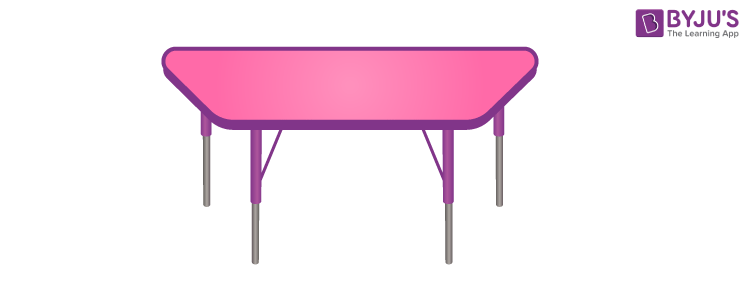## Types of Trapezium

The trapezium is categorised into three types:

• Isosceles Trapezium
• Scalene Trapezium
• Right Trapezium

### Isosceles trapezium

If the legs or the non-parallel sides of the trapezium are of equal length, then it is called an isosceles trapezium.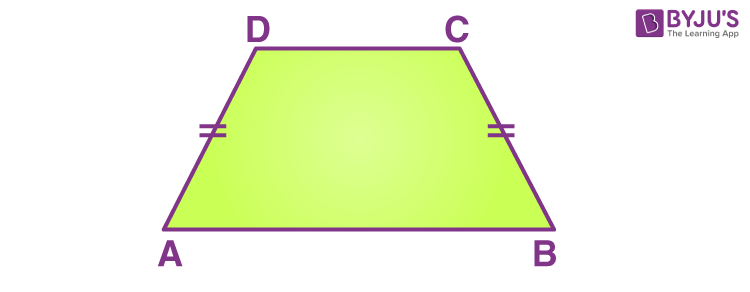### Scalene Trapezium

A trapezium with all the sides and angles of different measures is called a scalene trapezium.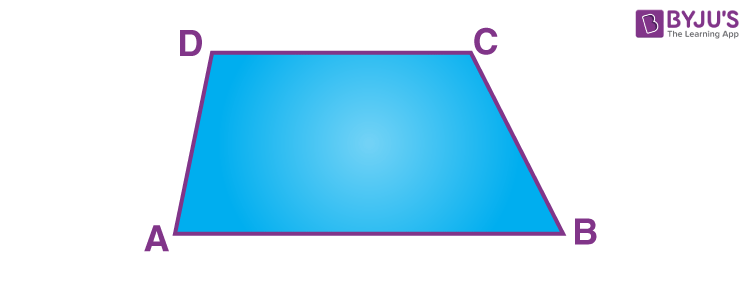### Right Trapezium

A right trapezium is a trapezium that has at least two right angles, adjacent to each other.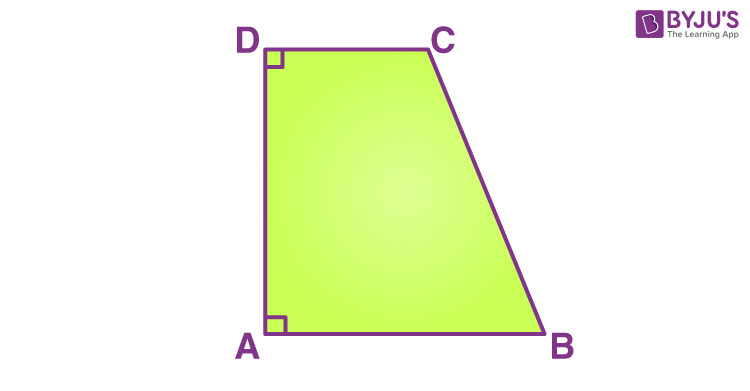## Irregular Trapezium

We know, a trapezium has exactly one pair of parallel sides and the other two sides are non-parallel. Now a regular trapezium will have the other two non-parallel sides equal, whereas an irregular trapezium will have the other two non-parallel opposite sides, unequal.

## Properties of Trapezium

Some important properties of a trapezium are as follows:

• In trapezium, exactly one pair of opposite sides are parallel
• The diagonals intersect each other
• The non-parallel sides in the trapezium are unequal except in isosceles trapezium
• The line that joins the mid-points of the non-parallel sides is always parallel to the bases or parallel sides which is equal to half of the sum of parallel sides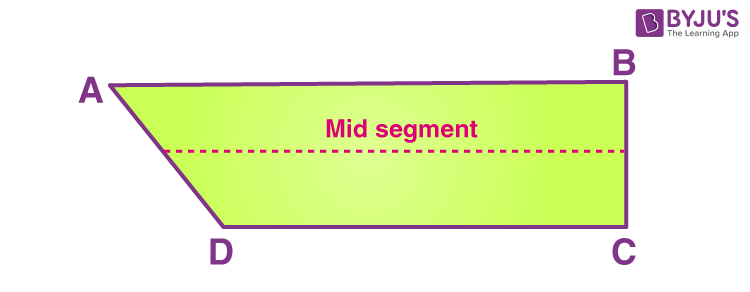Mid-segment = (AB+ CD)/2

Where AB and CD are the parallel sides or bases

• In isosceles trapezium, the legs or non-parallel sides are congruent
• The sum of the interior angles of the trapezium is equal to 360 degrees. i.e., ∠A + ∠B + ∠C + ∠D = 360°
• The sum of the two adjacent angles is equal to 180°. This means that the two adjacent angles are supplementary.

## Trapezium Formulas

The two important formulas of trapezium are given below:

 Area of Trapezium ½ (Sum of parallel side) (Distance between parallel sides) Perimeter of Trapezium Sum of all four sides

## Area of Trapezium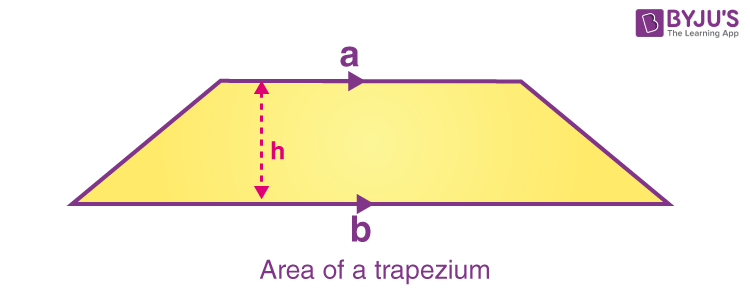The area of a trapezium can be found by taking the average of the two bases of a trapezium and multiply by its altitude. So, the area of the trapezium formula is given as:

 Area of a Trapezium, A = h(a+b)/2 square units.

Where,

“a” and “b” are the bases

“h” is the altitude or height.

### Area of Isosceles Trapezium

Let a and b are the length of parallel sides of a trapezium ABCD, such as a is the base length and b is the length of side parallel to a. Therefore,

a>b

Now, c is the length of two non-parallel sides and h is the height of an isosceles trapezium. So here,

AB = a, CD = b, BC = AD = c

If we draw perpendicular h from CD to meet AB at E, such that, it forms a right triangle, AED, then,

Length of perpendicular, h = √(c2 – (a-b)2)   [By Pythagoras theorem]

As we know, the formula for area of trapezium is:

Area = ½ h(a+b)

 Area of isosceles trapezium = 1/2 [√(c2 – (a-b)2) (a+b)]

## Perimeter of Trapezium

The perimeter of a trapezium is found by adding all the sides. Therefore, the perimeter of a trapezium formula is given as

The perimeter of Trapezium, P = a + b+ c + d units

Where,

“a, b, c, d” are the sides of the trapezium.

### Perimeter of Isosceles Trapezium

If a and b are the length of parallel sides and c is the length of two non-parallel sides, in an isosceles trapezium, then the perimeter will be:

Perimeter = a+b+2c

Example: If the length of parallel sides of a regular trapezium is 10 cm and 12 cm, and the length of non-parallel sides is equal to 5 cm each. Then find the perimeter.

Given, a=10 cm, b=12 cm and c= 5 cm

Therefore, using the perimeter formula, we get;

Perimeter = a+b+2c = 10+12+2(5)

P = 10+12+10

P = 32 cm

## Trapezium and Trapezoid

The trapezium is a four-sided polygon and a two-dimensional figure which has exactly one pair of parallel sides opposite to each other.

A trapezoid is also a four-sided polygon and with one pair of parallel sides opposite to each other. The parallel sides are the bases and another two sides are called legs of the trapezoid.

Although both words are used differently in different countries. But in general Mathematics, both are same.

## How to Find Angles of Trapezium

For a regular or isosceles trapezium, the sets of angles adjoined by parallel lines are equal. Also, we know, for any quadrilateral the sum of all the interior angles is equal to 360 degrees.

Hence, if an angle say, x, is given between the one parallel side and one non-parallel side, then subtracting twice of this angle from 360, will give the sum of two angles on the formed opposite side of x. Once, the sum is determined, then by dividing the sum by 2 will give the measure of the fourth angle.

Example: If a base angle of a trapezium, included between a parallel side and a non-parallel side is equal to 72 degrees. Then find the angle opposite to the given angle.

Given, one of the base angles of a trapezium say ABCD is 72 degrees.

Let the angle to be found = x

As we know, by angle sum property of quadrilateral, sum of all the angles equal to 360 degrees.

Now subtract 2 x 72 from 360,

360 – 144 = 216

Hence, 216 is the sum of the above two angles.

Since, both the above angles are equal for an isosceles trapezium, hence,

x = 216/2 = 108

Which is the required angle

## Solved Examples on Trapezium

Q.1: Find the area of the trapezium, in which the sum of the bases (parallel sides) is 60 cm and its height is 20 cm.

Solution: Given;

Let, Sum of the bases, (a+b) = 60 cm

Height, h = 20 cm

We know that,

Area of a Trapezium, A = h(a+b)/2 square units.

Substitute the given values,

A = (60/2)× 20

A = 30 × 20

A = 600 cm2.

Therefore, the area of trapezium = 600 cm2.

Q.2: Find the perimeter of a trapezium whose sides are 3cm, 4cm, 5cm and 6cm.

Solution: By the formula of the perimeter of trapezium, we know;

P = Sum of all the sides

P = 3+4+5+6

P = 18cm

Hence, the perimeter is 18cm.

## Practice Questions on Trapezium

1. PQRS is a trapezium in which PQ||SR and ∠P=130°, ∠Q=110°. Then what is the measure of ∠R?
2. In trapezium ABCD with AB||CD, if ∠A = 100°, then what is the measure of ∠D?
3. ABCD is a trapezium such that AB||CD, ∠A : ∠D = 2 :1, ∠B : ∠C = 7 : 5. Find the angles of the trapezium.

## Frequently Asked Questions – FAQs

Q1

### Does a Trapezium have Parallel Sides?

According to the definition of trapezium, it is a quadrilateral with one pair of parallel sides. Thus, a trapezium does have parallel sides.

Q2

### Are the Diagonals of a Trapezium Equal?

No, the diagonals of a trapezium may not be equal. For a trapezium, only 1 pair of its sides are parallel. But, for any quadrilateral to have equal diagonals, two pairs of sides should be parallel like in a square, rectangle, etc.

Q3

### Do Diagonals Bisect each other in a Trapezium?

No, the diagonals of a trapezium might not bisect each other. If the diagonals are bisecting, the trapezium will be a parallelogram. So, every parallelogram is a trapezium but every trapezium might not be a parallelogram.

Q4

### Is square a trapezium?

No, to be a trapezium or a trapezoid, a quadrilateral should have exactly one pair of parallel sides and the other two sides are non-parallel.

Stay tuned with BYJU’S – The Learning App to learn other interesting maths-related articles and also watch interactive videos to learn with ease.